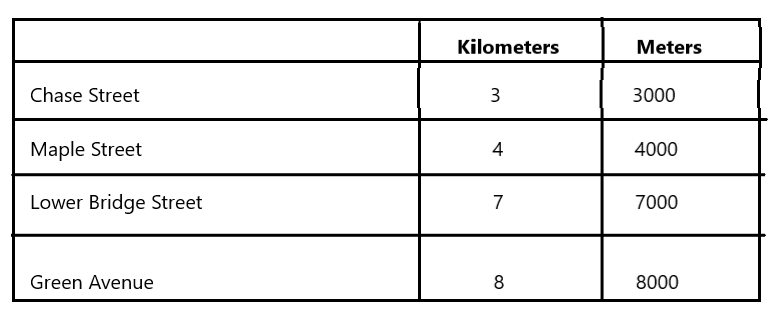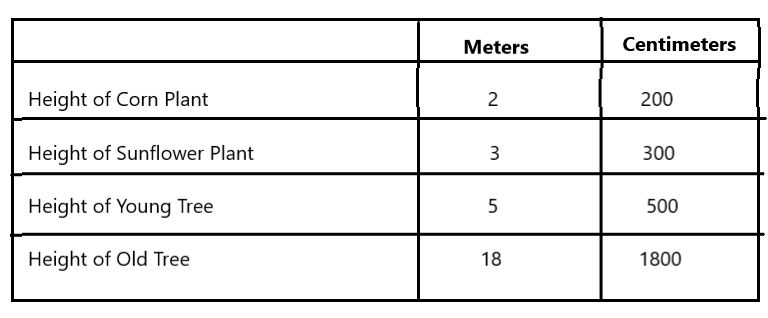# McGraw Hill Math Grade 4 Chapter 10 Lesson 2 Answer Key Using Tables with Metric Units of Length

Practice the questions of McGraw Hill Math Grade 4 Answer Key PDF Chapter 10 Lesson 2 Using Tables with Metric Units of Length to secure good marks & knowledge in the exams.

## McGraw-Hill Math Grade 4 Answer Key Chapter 10 Lesson 2 Using Tables with Metric Units of Length

Solve

Question 1.
Draw a table to show the following data. Then convert each length to meters.
Chase Street: 3 kilometers
Maple Street: 4 kilometers
Lower Bridge Street: 7 kilometers
Green Avenue: 8 kilometersExplanation:
I drew a table to show the given data
1 kilometer = 1000 meters
So, I converted each length from kilometers to meters.

Question 2.
Draw a table to show the following data. Then convert each length to centimeters.
Height of Corn Plant: 2 meters
Height of Sunflower Plant: 3 meters
Height of Young Tree: 5 meters
Height of Old Tree: 18 meters